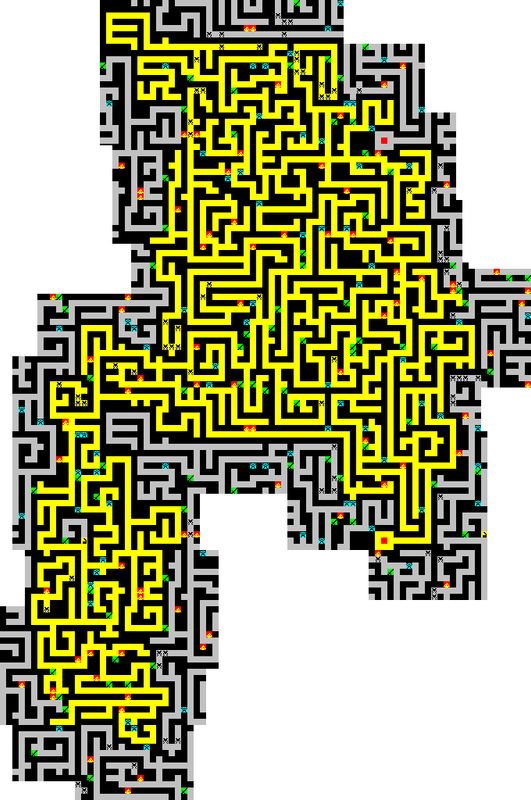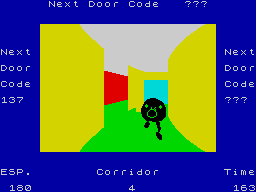# Looking for any maze game where the map is different every time

Hi folks,
I'm looking to sharpen my game writing skiils (or lack thereof!) and I need an example of a simple maze game which creates a different map to explore every time the game is played. I think the term is procedurally generated? The simpler the better. Ideally I'm after after a BASIC game with UDG characters/objects with the map viewed from above. I'm pretty sure the Spectrum has an example or two as I remember seeing them way back when but I can't recall any names to search for.

Cheers!

• maziacs
http://www.worldofspectrum.org/infoseekid.cgi?id=0003100

are there 'angband' clones on ZX ?
or Moria
https://en.wikipedia.org/wiki/Moria_(video_game)
Post edited by Crisis on
my old website http://home.hccnet.nl/c.born/ has changed to http://www.cborn.nl/zxfiles/ so just click it and select a file
• edited July 2019
Warlock of Firetop Mountain?

It’s not in basic, but the source of that, Halls and his other games are freely available on Simon’s site.
Post edited by colonel32 on
Robin Verhagen-Guest
SevenFFF / Threetwosevensixseven / colonel32
NXtel NXTP ESP Update ESP Reset CSpect Plugins
• Wheelie has randomly generated maps, but that's a side view.
• Fred
• I don't know if that's what you're looking for, but here is a simple 2D maze generator in Basic:```   10 LET ml=5
20 INK 0: PAPER 0: BORDER 0: CLS
30 INK 1: PAPER 1
40 DIM x(4)
50 DIM y(4)
60 LET x(1)=1: LET y(1)=0
70 LET x(2)=0: LET y(2)=-1
80 LET x(3)=-1: LET y(3)=0
90 LET x(4)=0: LET y(4)=1
100 PRINT AT 0,0;"###############################";
110 FOR i=1 TO 19: PRINT AT i,0;"#";AT i,30;"#";: NEXT i
120 PRINT AT 20,0;"###############################";
130 LET r=126
140 LET xp=2+2*INT (RND*14)
150 LET yp=2+2*INT (RND*9)
160 IF SCREEN\$ (yp,xp)<>" " THEN GO TO 140
170 LET l=0
180 PRINT AT yp,xp;"#";
190 LET r=r-1
200 REM PRINT #1;AT 0,0;r;" ";
210 LET l=l+1
220 IF l>=ml THEN GO TO 360
230 LET d=INT (RND*4)+1
240 FOR j=1 TO 4
250 IF SCREEN\$ (yp+2*y(d),xp+2*x(d))=" " THEN GO TO 300
260 LET d=d+1
270 IF d>4 THEN LET d=1
280 NEXT j
290 GO TO 360
300 LET yp=yp+y(d)
310 LET xp=xp+x(d)
320 PRINT AT yp,xp;"#";
330 LET yp=yp+y(d)
340 LET xp=xp+x(d)
350 GO TO 180
360 IF r>0 THEN GO TO 140
370 INK 0: PAPER 7
380 PAUSE 0
```
• That's beyond me - I read the Wikipedia article but it just passed across my brain unprocessed! My pathetic math skills conspire to prevent me from understanding the algorithm but I DID get the divide by two and insert gaps way of doing it - that I think I could manage to program.

I have nothing but sincere admiration for the real programmers here, I always try to get a sense of what folks are discussing but its often over my head.
• Many thanks for the links and info, there's plenty to keep me busy.
• One thing I've always wondered about : Does the Maziacs map go on forever, or wrap around, or is somehow finite and always ends?

Otherwise, Halls Of The Things is the goto game for maps. I'm fairly certain thats a Recursive Backtracker generator with some extra holes poked in the walls if two cells are in a short contiguous distance of each other.
• If you really want to dig into generating mazes then I’d recommend this book (although obviously it’s talking about maze algorithms to be applied to the language of your choice, not specifically for BASIC or Z80):

https://www.amazon.co.uk/Mazes-Programmers-Twisty-Little-Passages/dp/1680500554/ref=sr_1_1?crid=1AEAR9Z618VKU&keywords=mazes+for+programmers&qid=1562667437&s=gateway&sprefix=Mazes+for+pro,aps,163&sr=8-1
• The Oracle's Cave map is randomly generated but not a maze game though.
• Simon Goodwin's type-in maze game "Runaway Robot" is in the Games Computing section at The Type Fantastic.

""The heart of Runaway Robot is the random circuit generator, which produces an almost infinite number of different mazes. In each maze you are guaranteed that a path will exist between every point, so you can never end up "trapped", however complicated the maze.""
• Good old Alcatraz Harry - it's a flick screen game but it's generated randomly each time (I think). Written in BASIC too.

those-funny-games.blogspot.com/2013/03/funny-games-alcatraz-harry-classic-zx.html

• morph wrote: »
One thing I've always wondered about : Does the Maziacs map go on forever, or wrap around, or is somehow finite and always ends?.

I wondered that too, so I made a map.It wraps around.
I've highlighted all the bits you can reach in yellow. The gold is on the left-hand side, quite near the bottom, but you can also see it on the right, just a little way away from the start/finish place.

(Yes, I've just spent ages making a map of a game which is different every time you play. Pointless really, but interesting.)
Thanked by 1morph
• On a related note, does anyone know of a 3d maze game where you're chased by a green monster? I'm sure I've seen it in the WoS archive somewhere. I remember it being similar to 3D Monster Maze?
• @slomp
Thank you!
Could you explain a bit how I can adapt it to keep corridors 2 characters apart?

I'm not great at explaining things, but I'll give it a shot. I hope you're okay with me replying in the thread and not per PM, but I feel if I put in the work it might as well help others.

Take a piece of graph paper, draw a box on it. Then take a pen put it on any empty crossing (where 2 lines intersect) inside the box draw a line to an empty neighbor crossing. Repeat until there are no empty neighbor crossings or your wall has reached a maximum length (ml). Then repeat with the next empty crossing until no empty crossings are left. Your maze is done.

To translate this to the screen you just have to take a cell as the center of the crossing and draw the walls according to your desired corridor width. So if you want 1 cell wide corridors you move 2 cells, if you want 2 cell wide corridors you move 3 cells etc.

I have tried to adapt the program to your needs, but as usual Basic programs are pretty hard to read, even if you wrote them yourself a few months ago.
```   10 LET ml=5
20 INK 0: PAPER 0: BORDER 0: CLS
30 INK 1: PAPER 1
40 DIM x(4)
50 DIM y(4)
60 LET x(4)=1: LET y(4)=0
70 LET x(1)=0: LET y(1)=-1
80 LET x(2)=-1: LET y(2)=0
90 LET x(3)=0: LET y(3)=1
100 PRINT AT 0,0;"###############################";
110 FOR i=1 TO 20: PRINT AT i,0;"#";AT i,30;"#";: NEXT i
120 PRINT AT 21,0;"###############################";
130 LET r=54
140 LET xp=3+3*INT (RND*9)
150 LET yp=3+3*INT (RND*6)
160 IF SCREEN\$ (yp,xp)<>" " THEN GO TO 140
170 LET l=0
180 PRINT AT yp,xp;"#";
190 LET r=r-1
200 PRINT #1;AT 0,0;r;" ";
210 LET l=l+1
220 IF l>=ml THEN GO TO 990
230 LET d=INT (RND*4)+1
240 FOR j=1 TO 4
250 IF SCREEN\$ (yp+3*y(d),xp+3*x(d))=" " THEN GO TO 300
260 LET d=d+1
270 IF d>4 THEN LET d=1
280 NEXT j
290 GO TO 990
300 LET yp=yp+y(d)
310 LET xp=xp+x(d)
320 PRINT AT yp,xp;"#";
330 LET yp=yp+y(d)
340 LET xp=xp+x(d)
350 PRINT AT yp,xp;"#";
360 LET yp=yp+y(d)
370 LET xp=xp+x(d)
500 GO TO 180
990 IF r>0 THEN GO TO 140
1000 INK 0: PAPER 7
```

Also I have just noticed that I derived this from an older version of the code, so that might make it a little harder to spot the changes. Sorry for that.

If you have more questions, I'm happy to help you as best as I can.
Thanked by 1slomp
• LevelUp wrote: »
On a related note, does anyone know of a 3d maze game where you're chased by a green monster? I'm sure I've seen it in the WoS archive somewhere. I remember it being similar to 3D Monster Maze?

Corridors Of Genon? The monster isn't green but the floor is.• Or Escape from New Generation - although that was more of a 3d pacman overhead look
No one important.
• If we're talking impenetrable maze generators, I like this one:
```10 REM Maze Generator
20 LET mw=99: LET mh=59: GO SUB 100
30 LET m\$="ª"*mw*mh: LET b\$="1"*mw+(("1"+(" "*(mw-2))+"1")*(mh-2))+("1"*mw): LET cc=mw+2: DIM of=-1,-mw,1,mw: LET m\$(cc)=" ": LET stk\$=QCHR\$ cc: DIM nb(4)
40 LET nn=0: FOR n=1 TO 4: IF b\$(cc+of(n))<>"1" THEN IF m\$(cc+(of(n)*2))<>" " THEN INC nn: LET nb(nn)=n
50 NEXT n
60 IF nn>0 THEN LET o=of(nb(INT(RND*nn)+1)): LET m\$(cc+o)=" ": LET m\$(cc+(o*2))=" ": GO SUB 1000: LET stk\$=(QCHR\$(cc) AND nn>1)+stk\$: INC cc,o*2: GO TO 40
70 IF stk\$<>"" THEN LET cc=QCODE stk\$: LET stk\$=stk\$(5 TO): GO TO 40
80 PAUSE 0: RUN
90 LET c=cc+o: PRINT AT c DIV mw,c MOD mw;" ": LET c=cc+o*2: PRINT AT c DIV mw,c MOD mw;" ": RETURN
100 FOR x=1 TO mw: FOR y=0 TO mh-1: PRINT AT y,x;"ª": NEXT y: NEXT x: PRINT AT 1,2;" ": RETURN
```

Runs on SpecBAS, but shouldn't be too hard to convert to a Speccy.• Now that's a Maze worthy of Maze Craze (Atari VCS game, great fun). Maziacs was my second favorite maze game, both featuring proper "single route to any location" type mazes

It's on my bucket list to try to write an Spectrum based update of Maze Craze, with even better graphics ;)

I might need to nab that knotty list, and travel to unravel it and turn it to assembler.

There's some nice description of Maze generation methods here https://www.jamisbuck.org/mazes/ and it also neatly shows the mazes being generated